#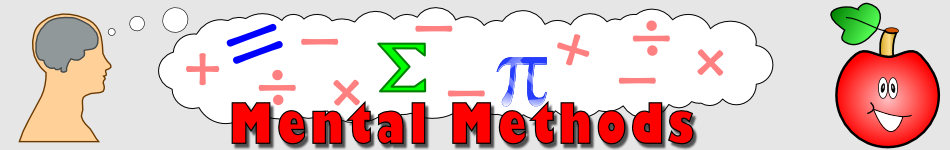There are 366 different Starters of The Day, many to choose from. You will find below some starters on the topic of Mental Methods. A lesson starter does not have to be on the same topic as the main part of the lesson or the topic of the previous lesson. It is often very useful to revise or explore other concepts by using a starter based on a totally different area of Mathematics.

Main Page

### Mental Methods Starters:Develop a quick way of mentally multiplying any number by 1001.A game based around the concept of factors and abundant numbers.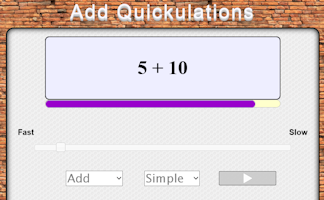Calculations appear on the screen every few seconds. This mental arithmetic starter provides pace to the start of the Maths lesson.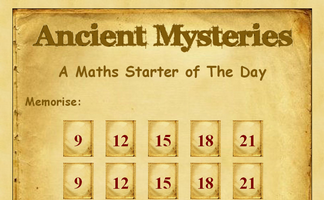This activity requires students to memorise fifteen numbers in a three by five grid.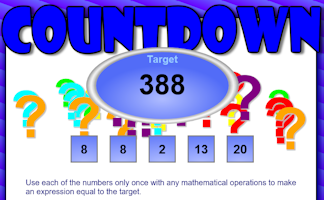How close can you get to the target by making a calculation from the five numbers given?Random division calculations appear on screen every few seconds.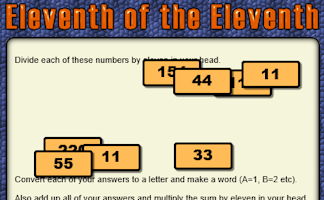Fit families onto eleven seater buses without splitting up the families.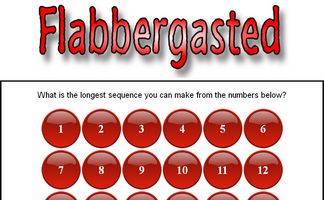If each number in a sequence must be a factor or multiple of the previous number what is the longest sequence that can be made from the given numbers?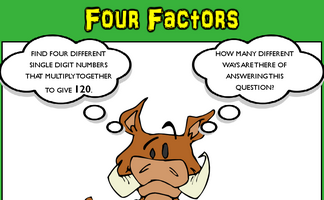Find four single digit numbers that multiply together to give 120. How many different ways are there of answering this question?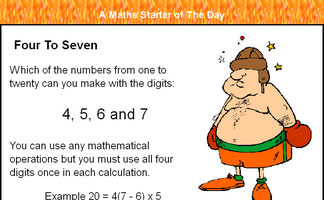Which of the numbers from one to twenty can you make with the digits 4, 5, 6 and 7?Convert fractions to decimals, decimals to percentages and percentages to fractions.Without a calculator perform some calculations requiring a knowledge of place value.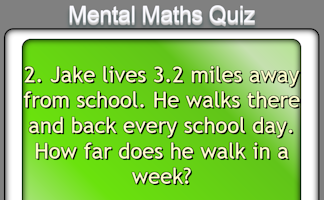A traditional twenty question mental arithmetic test presented as a PowerPoint presentation.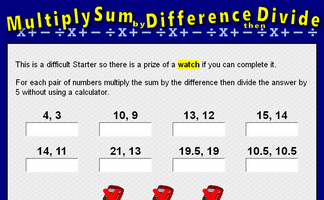For each pair of numbers multiply the sum by the difference then divide the answer by 5.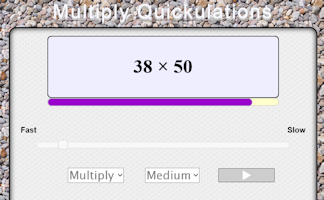Random multiplications appear on screen every few seconds.For each pair of numbers subtract the sum from the product then divide the result by 20 without a calculator.Find which numbers in a given list do not combine with other numbers on the list to make a given sum.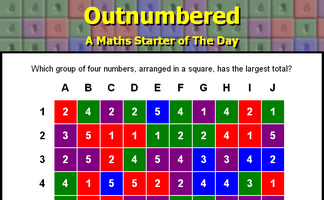Which group of four numbers, arranged in a square, has the largest total?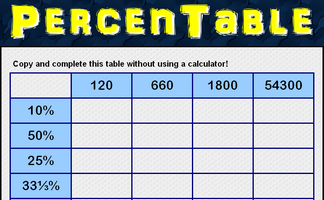Complete the table by calculating common percentages without using a calculator.Stand at the point between the classroom walls to represent a given number.It is called Refreshing Revision because every time you refresh the page you get different revision questions.An activity involving a calculator which is missing the six button. Can you evaluate the given expressions without using the six?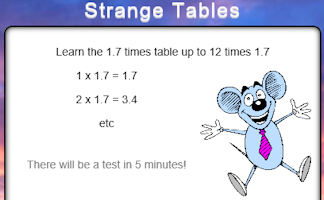A challenge to learn an unfamiliar times table involving decimals.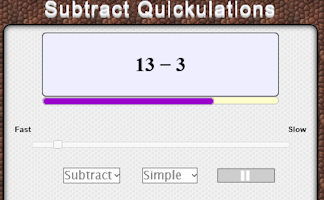Calculations appear on the screen every few seconds.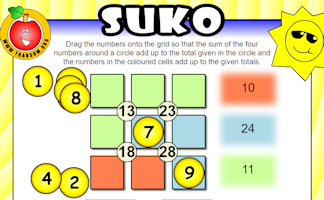Interactive number-based logic puzzle similar to those featuring in The Times and Telegraph newspapers.Learn an unusual times table from the strategic finger moving up and down the 'Table Leg'!Multiply the number on the spider's back by the numbers next to its legs.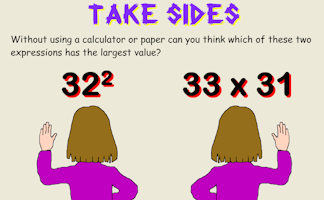Put up your right hand or left hand depending on the expressions that appears.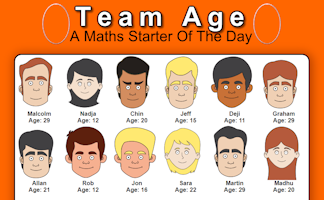Work out who is in which team from the information given.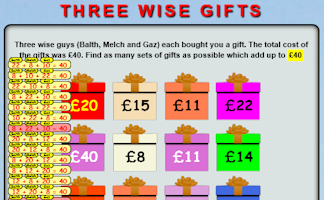Find as many sets of three of the available numbers as possible which add up to the given total.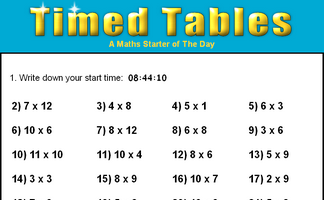How fast can you answer 24 mixed times tables questions?Complete the sums using only the given numbers then check your calculations are correct.Find as many sets of three of the available numbers as possible which add up to the given total.Find five integers that multiply together to give a product of twelve.

## Exercises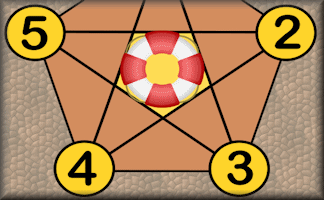#### 23 or Bust

A game involving mental arithmetic and strategy for two players or one player against the computer.#### Beat The Clock

It is a race against the clock to answer 30 mental arithmetic questions. There are nine levels to choose from.#### Bidmaze

Find your way through the maze encountering mathematical operations in the correct order to achieve the given total.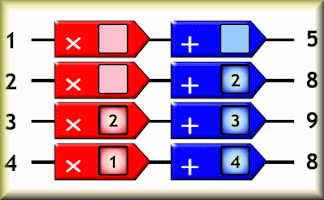#### Brainbox

A puzzle requiring the arrangement of numbers on the function machines to link the given input numbers to the correct output.#### Chain Challenge

How fast can you perform all of the given operations without using a calculator?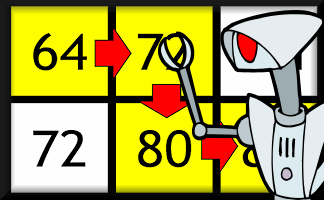#### Convoluted

Find the runs of four multiples in order as quickly as you can.#### Digital Darts

An online darts game for one or two players requiring skill, strategy and mental arithmetic.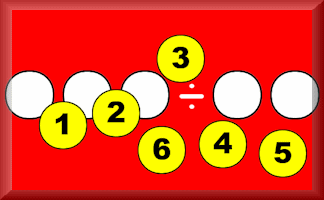#### Digivide

Arrange the numbers from 1 to 6 in the spaces to make the division calculation correct.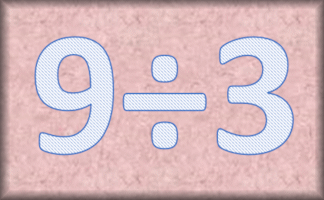#### Divides Exactly

Find which divisions result in whole number quotients. A mental arithmetic speed challenge.#### Divisibility Test

Practise using the quick ways to spot whether a number is divisible by the digits two to nine.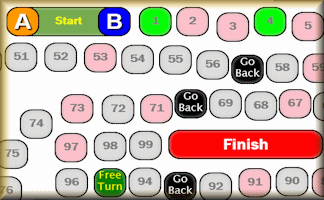#### Dump-A-Dice Race

An online board game for two players involving prime and square numbers and making choices.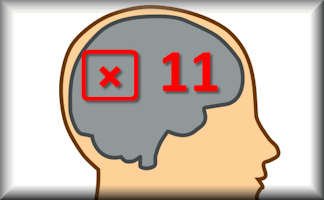#### Estimation Golf

Play a round of golf using your estimation skills rather than golf clubs.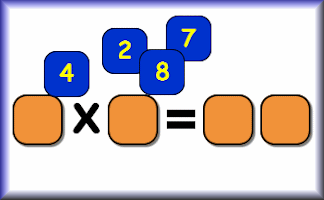#### Expedite

Drag the numbered cards to produce a multiplication fact. Complete twenty mixed times tables questions to earn a trophy.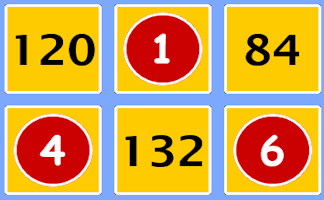#### Fast Factors

How quickly can you arrange the cards to match the times tables?#### Flash Tables

A never ending sequence of times tables questions to be projected on to a whiteboard or screen.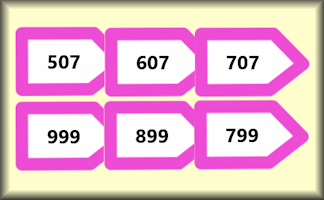#### Furthermore

A self-marking exercise on counting forwards or backwards in tens, hundreds, thousands etc.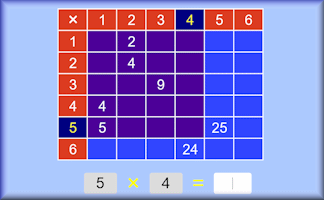#### Grid Arithmetic

Fill in a multiplication grid with the answers to simple multiplication and division questions.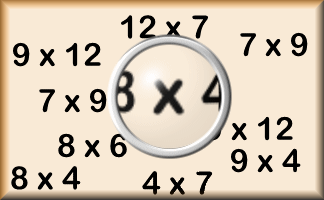#### Hard Times

The hardest multiplication facts (according to Transum research) are presented in the form of pairs games.#### Just In Time

Every 10 seconds a new calculation appears on the screen: A dynamic visual aid.Without a calculator perform some calculations requiring a knowledge of place value.#### Latin Square Puzzles

Arrange the given digits to make a Latin square with the given row and column calculation results.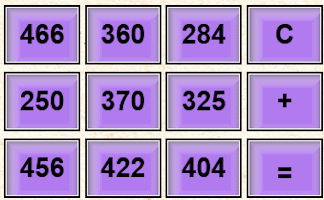#### Make 1000

Use the numbers on the strange calculator to make a total of 1000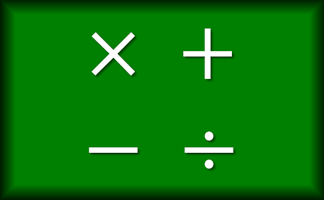For each pair of numbers subtract the sum from the product then divide the result by 20 without a calculator.#### Mental Strategies

Practise your mental arithmetic skills and learn some new strategies with this self marking exercise.#### Mixpressions

Arrange the cards to create a valid mathematical statement.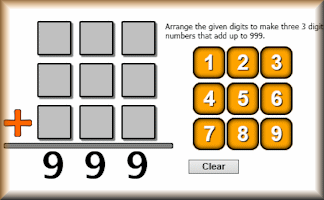#### Nine Nine Nine

Use the digits 1 to 9 to make three 3 digit numbers which add up to 999.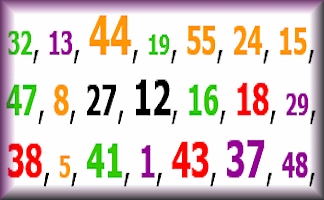#### No Partner

Find which numbers in a given list do not combine with other numbers on the list to make a given sum.#### Numbasics

A daily workout strengthening your ability to do the basic mathematical operations efficiently.#### Number Crunch Saga

A lively numeracy game requiring you to align three numbers to create the given target sum or product.#### Number Skills Inventory

A checklist of basic numeracy techniques that every pupil should know.#### Numskull

Interactive, randomly-generated, number-based logic puzzle designed to develop numeracy skills.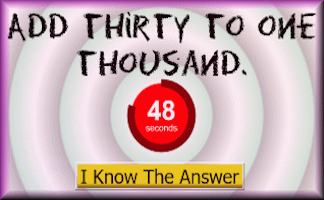#### One Minute Maths

A challenge to mentally add numbers together without making the classic place value mistakes.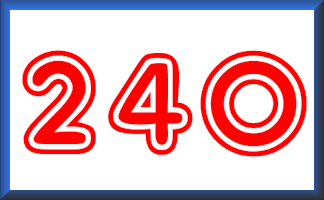#### Pairs 240

Find the pairs of numbers that multiply together to give a product of 240 in this collection of matching games.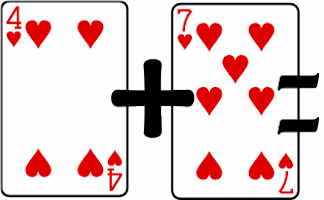#### Pairs Eleven

Find pairs of playing cards of the same suit which add up to eleven.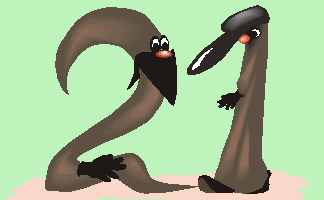#### Pairs Twenty One

Find the pairs of numbers that add up to 21 in this collection of matching games.#### Percentages

Practise calculating simple percentages in your head with this animated visual aid.#### Perfect Magic Square

Arrange the sixteen numbers on the four by four grid so that groups of four numbers in a pattern add up to the same total.#### Pesto

Students classify numbers randomly appearing on the screen by holding up cards#### Pick The Primes

Pick the prime fruit from the tree as quickly as possible. Practise to improve your personal best time.#### Playing Card Maths

Imagine you are on a desert island with nothing but a pack of playing cards. Do you have to stop learning mathematics?#### Powten

Practise multiplying and dividing by powers of ten without using a calculator.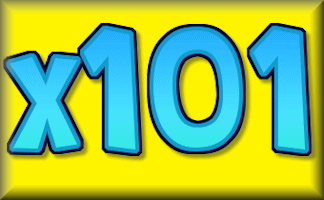#### Quick

Can you multiply a number by 1001 in your head? This exercise provides practice in this and other similar challenges.#### Quickulations

A mental arithmetic visual aid that displays random calculations then after a few seconds displays the answers.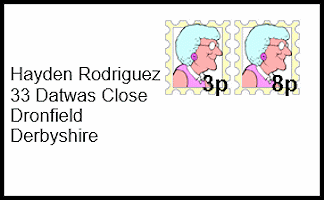#### Stamp Sticking

Drag stamps onto the envelopes to make the exact postage as shown at the top left of each envelope.#### Sum Game

A game against the clock to find the numbers which add up to the target number.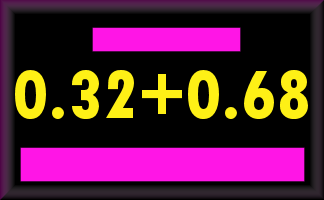#### Sum to One

Find pairs of numbers that add up to one.#### TablesMaster#### Three Ways

Find three different ways of multiplying four different digits together to get the given target number. There are nine levels for this online challenge.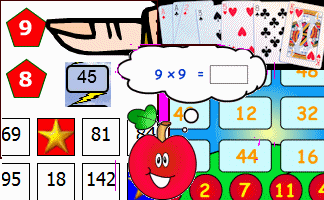#### Times Tables#### Triplets

Find as many sets of three of the available numbers as possible which add up to the given total.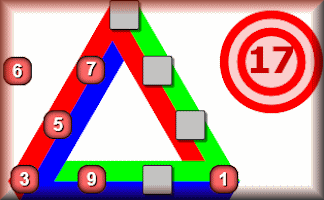#### Triside Totals

Arrange the digits 1 to 9 on the triangle so that the sum of the numbers along each side is equal to the given total.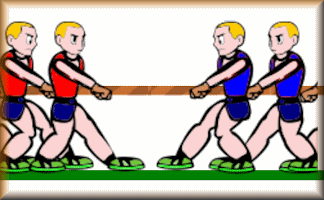#### Tug of War

A game for two players or teams testing their speedy reactions to mental arithmetic questions.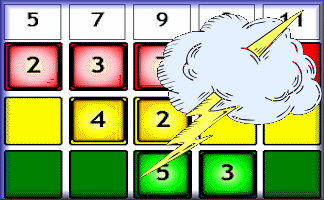#### Zygo

Interactive, randomly-generated, number-based logic puzzle designed to develop numeracy skills.

### Search

The activity you are looking for may have been classified in a different way from the way you were expecting. You can search the whole of Transum Maths by using the box below.

Have today's Starter of the Day as your default homepage. Copy the URL below then select
Tools > Internet Options (Internet Explorer) then paste the URL into the homepage field.

Set as your homepage (if you are using Internet Explorer)

Do you have any comments? It is always useful to receive feedback and helps make this free resource even more useful for those learning Mathematics anywhere in the world. Click here to enter your comments.For All: